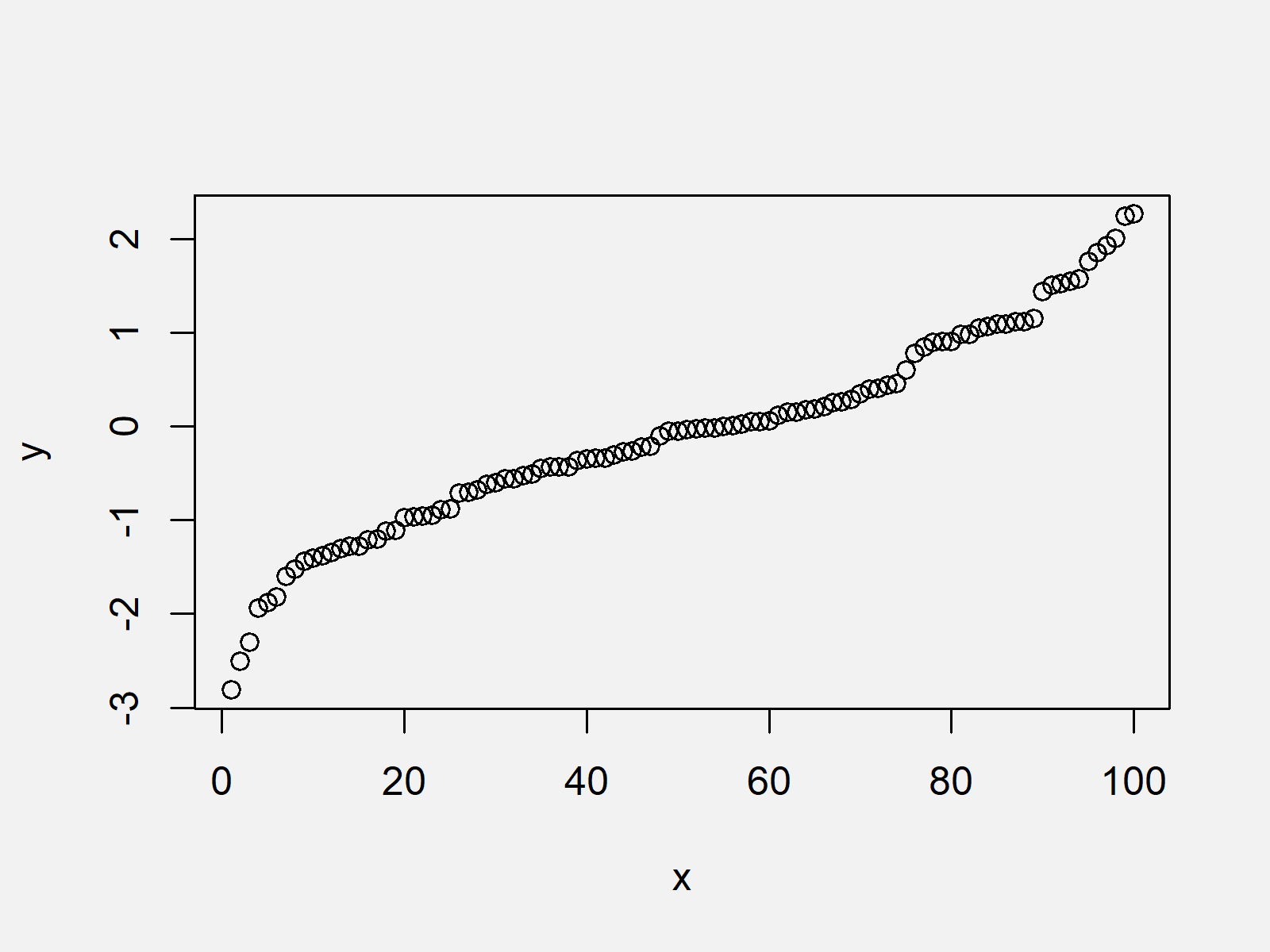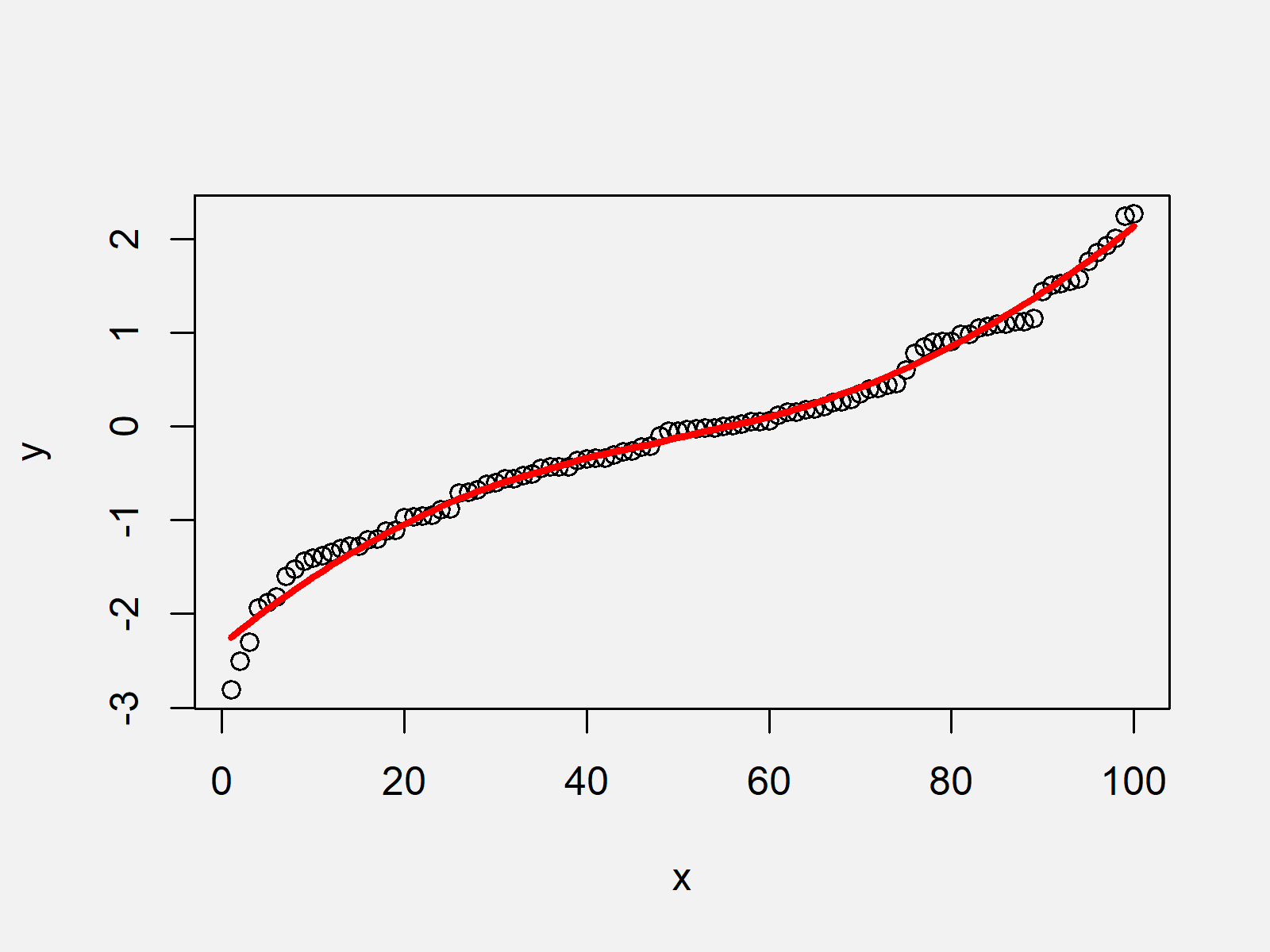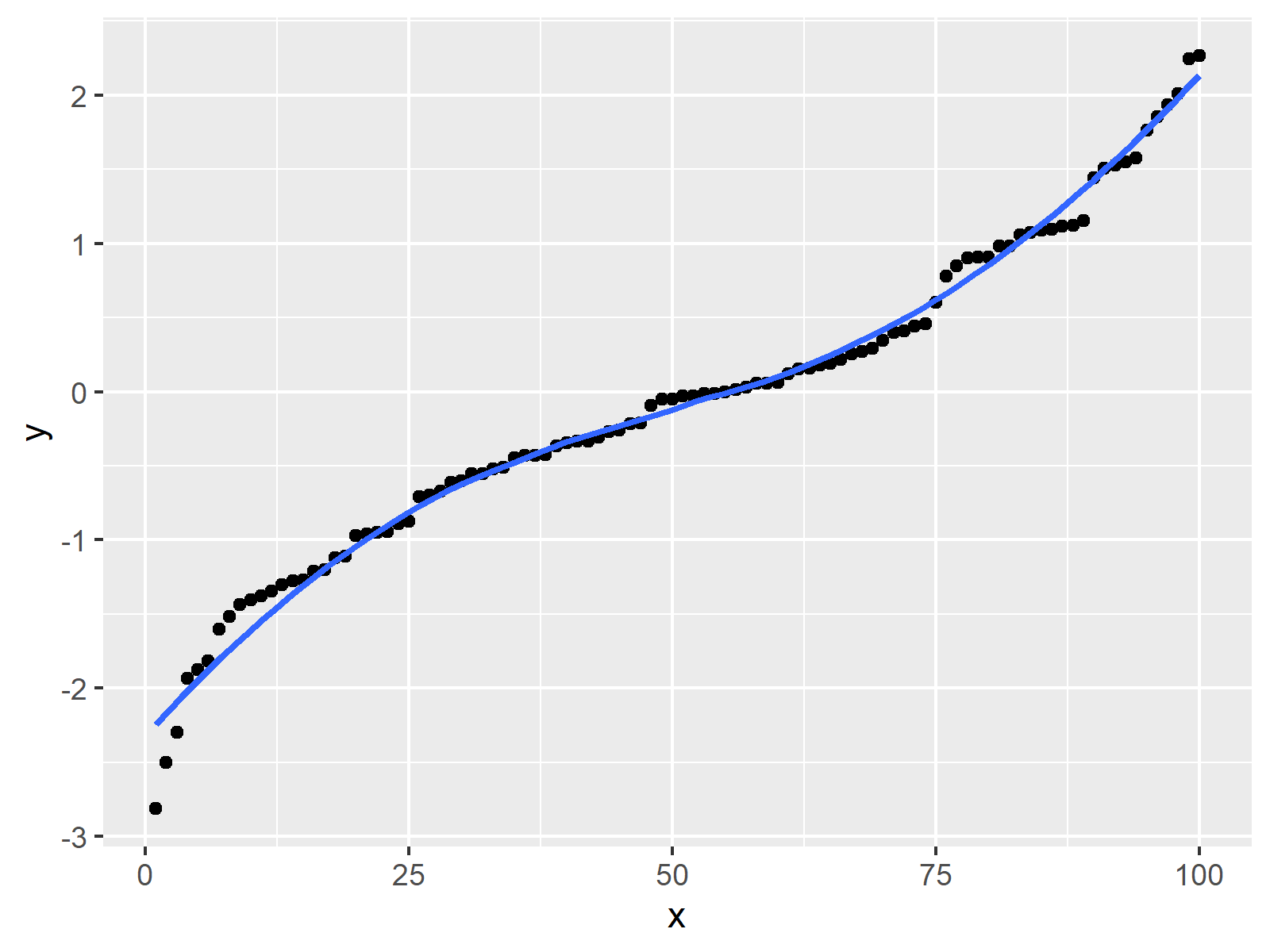# Fit Smooth Curve to Plot of Data in R (Example)

In this tutorial you’ll learn how to draw a smooth line to a scatterplot in the R programming language.

Here’s how to do it:

## Introduction of Example Data

The following data is used as basement for this R programming tutorial:

```set.seed(87564832)              # Create example data
x <- 1:100
y <- sort(rnorm(100))```

The previous output of the RStudio console shows the structure of our example data. It consists of two numeric vectors each containing 100 values.

Now, we can draw our data as follows:

`plot(x, y)                      # Plot without line`Figure 1 visualizes the output of the previous R syntax: A scatterplot showing our data. However, this scatterplot does not show a fitted curve yet…

## Example 1: Creating Scatterplot with Fitted Smooth Line Using Base R

The following R code explains how to draw a fitted curve to our example plot. Have a look at the following R code:

`curve_values <- loess(y ~ x)    # Apply loess function`
```plot(x, y)                      # Plot with line
lines(predict(curve_values),
col = "red",
lwd = 3)```As shown in Figure 2, we created a scatterplot with a fitted curve with the previous R code.

## Example 2: Creating Scatterplot with Fitted Smooth Line Using ggplot2 Package

Alternatively to Base R (as explained in Example 1), we can also use the ggplot2 package to draw a scatterplot with a fitted curve.

For this, we first have to store our data vectors in a data frame object as shown below:

```data_ggp <- data.frame(x, y)    # Store values in data frame
# x         y
# 1 1 -2.813293
# 2 2 -2.502170
# 3 3 -2.297299
# 4 4 -1.934714
# 5 5 -1.875186
# 6 6 -1.816849```

Furthermore, we have to install and load the ggplot2 package:

```install.packages("ggplot2")     # Install & load ggplot2
library("ggplot2")```

Next, we can use the geom_smooth function to add a fitted curve on top of our scatterplot as shown below:

```ggplot(data_ggp, aes(x, y)) +   # Draw ggplot2 scatterplot with smooth curve
geom_point() +
geom_smooth(se = FALSE,
method = "loess",
formula = y ~ x)```The previously shown figure visualizes the output of the previous R code – A ggplot2 scatterplot with smooth curve on top.

## Video & Further Resources

Please accept YouTube cookies to play this video. By accepting you will be accessing content from YouTube, a service provided by an external third party.If you accept this notice, your choice will be saved and the page will refresh.

Furthermore, you may have a look at the related R tutorials of my website. Some posts are shown below.

Subscribe to the Statistics Globe Newsletter

•Lara
June 16, 2023 3:16 pm

Hi Joachim,

thank you for your detailed explanation! Is there a way to find out the equation of this curve? I actually have some values predicting the probability of a certain outcome (logistic regression has been performed previously). By using this variables, I want to fit a curve and use it to estimate the risk for this outcome for every single of my patients. This would be done by putting the predicting variables in the equation, right?
•June 19, 2023 7:32 am